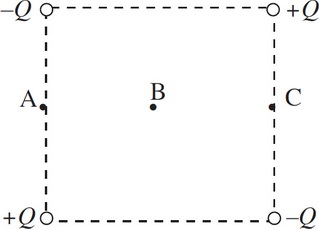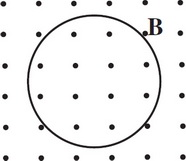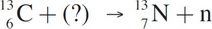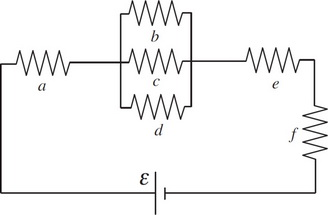# AP Physics 2 Practice Test 2

### Test Information10 questions18 minutes

1. Question below refers to the following plane diagram. The figure shows four point charges arranged at the corners of a square with point B as its center.At which points is the electric potential equal to zero?

2. A spherical balloon filled with helium is floating in air. If the balloon is inflated until its radius is doubled, how will the buoyant force on the balloon be affected?

3. A beam of monochromatic light entering a glass window pane from the air will experience a change in

4.A metal loop of wire shown above is situated in a magnetic field B pointing out of the plane of the page. If B decreases uniformly in strength, the induced electric current within the loop is

5. How does λe, the de Broglie wavelength of an electron (mass = me), compare with λp, the de Broglie wavelength of a proton (mass = mp) moving with the same kinetic energy as the electron?

6. Data is collected in an experiment preformed on an ideal gas. In the experiment, temperature (in K) is the independent variable and volume (in m3) is the dependent variable. If a plot is produced where the dependent variable is on the vertical axis, which of the following is true about the slope and y-intercept of the graph?

7. Which of the following changes to a double-slit interference experiment would increase the widths of the fringes in the interference pattern that appears on the screen?

8.Identify the missing particle in the nuclear reaction given above.

9. Question below refers to the circuit shown below:If each of the resistors in the circuit has a resistance of 3Ω, what must be the emf of the battery if the circuit draws a total current of 3 A ?

10. Question below refers to the circuit shown below:Which of the following relates Pb, the rate at which energy is dissipated by Resistor b, and PE, the rate at which energy is dissipated by Resistor E ?